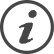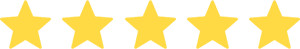### Discounted Cash Flow (DCF) Valuation Model

#### 8 Excel sheets | 15 Powerpoint slides

Easily estimate the value of a Business by reusing this Discounted Cash Flow (DCF) Valuation Model Template. The Excel Template was created by ex-Deloitte & McKinsey Consultants and Venture Capitalists. Don’t reinvent the wheel. Download this Template in a couple of clicks.## Content Details

Expand all
I. A DCF Valuation Model Template in Excel including:
1. An Income Statement
2. A Cash Flow Statement
3. A Balance Sheet Statement
4. A Discounted Cash Flow Model
5. Many Formulas to automatically calculate the Unlevered Free Cash Flow, the WACC, and Terminal Value
6. A real life example
7. 3 scenarios with adjustable assumptions automatically impacting the Financial Statements and Valuation
8. Links between the 3 Financial Statements and the DCF Model
II. A Step by Step Tutorial:
1. Estimate the Total Unlevered Free Cash Flow
2. Calculate the Total Net Present Value
3. Calculate the Weighted Cost of Capital
4. Calculate the Terminal Value
5. Calculate the Total Valuation